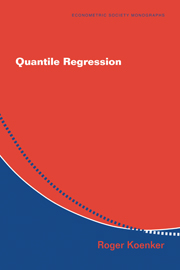Skip to content
Look Inside# Quantile Regression

## £32.99

• Date Published: July 2005
• availability: Available
• format: Paperback
• isbn: 9780521608275

## £32.99 Paperback

### Looking for an inspection copy?

This title is not currently available on inspection

Description
Product filter button
Description
Contents
Resources
Courses
• Quantile regression is gradually emerging as a unified statistical methodology for estimating models of conditional quantile functions. By complementing the exclusive focus of classical least squares regression on the conditional mean, quantile regression offers a systematic strategy for examining how covariates influence the location, scale and shape of the entire response distribution. This monograph is the first comprehensive treatment of the subject, encompassing models that are linear and nonlinear, parametric and nonparametric. The author has devoted more than 25 years of research to this topic. The methods in the analysis are illustrated with a variety of applications from economics, biology, ecology and finance. The treatment will find its core audiences in econometrics, statistics, and applied mathematics in addition to the disciplines cited above.

• First comprehensive study of quantile regression methods
• Tutorial on associated statistical software in R
• Illustrative applications from a broad variety of disciplines

## Reviews & endorsements

'… well written and easy to read, with useful illustrations of important aspects of quantile regression. It is obvious that the author knows the subject inside out, giving an up-to-date, exhaustive account of the subject. … The book is a valuable contribution to the statistical literature, and a must have for every statistician or econometrician interested in quantile regression methods.' Journal of the Royal Statistical Society

'It is well written and easy to read, with useful ilustrations of important aspects of quantile regression … a valuable contribution to the statistical literature and is essential for every statistician or econometrician who is interested in quantile regression methods.' Andreas Karlsson, Uppsala University

See more reviews

## Customer reviews

### Not yet reviewed

Be the first to review

Review was not posted due to profanity

×

## , create a review

(If you're not , sign out)

Your review must be a minimum of 12 words.

×

## Product details

• Date Published: July 2005
• format: Paperback
• isbn: 9780521608275
• length: 366 pages
• dimensions: 229 x 150 x 25 mm
• weight: 0.54kg
• contains: 63 b/w illus. 13 tables 20 exercises
• availability: Available

Part I. Introduction:
1. Means and ends
2. The first regression: an historical prelude
3. Quantiles, ranks, and optimization
4. Preview of quantile regression
5. Three examples
6. Conclusion
Part II. Fundamentals of Quantile Regression:
7. Quantile treatment effects
8. How does quantile regression work?
9. Robustness
10. Interpreting quantile regression models
11. Caution: quantile crossing
12. A random coefficient interpretation
13. Inequality measures and their decomposition
14. Expectiles and other variations
15. Interpreting misspecified quantile regressions
16. Problems
Part III. Inference for Quantile Regression:
17. The finite sample distribution of regression quantiles
18. A heuristic introduction to quantile regression asymptotics
19. Wald tests
20. Estimation of asymptotic covariance matrices
21. Rank based Inference for quantile regression
22. Quantile likelihood ratio tests
23. Inference on the quantile regression process
24. Tests of the location/acale hypothesis
25. Resampling methods and the bootstrap
26. Monte-Carlo comparison of methods
27. Problems
Part IV. Asymptotic Theory of Quantile Regression:
28. Consistency
29. Rates of convergence
31. Nonlinear quantile regression
32. The quantile regression rankscore process
33. Quantile regression asymptotics under dependent conditions
34. Extremal quantile regression
35. The method of quantiles
36. Model selection, penalties, and large-p asymptotics
37. Asymptotics for inference
38. Resampling schemes and the bootstrap
39. Asymptotics for the quantile regression process
40. Problems
Part V. L-Statistics and Weighted Quantile Regression:
41. L-Statistics for the linear model
42. Kernel smoothing for quantile regression
43. Weighted quantile regression
44 Quantile regression for location-scale models
45. Weighted sums of p-functions
46. Problems
Part VI. Computational Aspects of Quantile Regression:
47. Introduction to linear programming
48. Simplex methods for quantile regression
49. Parametric programming for quantile regression
50 Interior point methods for canonical LPs
51. Preprocessing for quantile regression
52. Nonlinear quantile regression
53. Inequality constraints
54. Weighted sums of p-functions
55. Sparsity
56. Conclusion
57. Problems
Part VII. Nonparametric Quantile Regression:
58. Locally polynomial quantile regression
59. Penalty methods for univariate smoothing
60. Penalty methods for bivariate Smoothing
61. Additive models and the Role of sparsity
Part VIII. Twilight Zone of Quantile Regression:
62. Quantile regression for survival data
63. Discrete Response models
64. Quantile autoregression
65. Copula functions and nonlinear quantile regression
66. High breakdown alternatives to quantile regression
67. Multivariate quantiles
68. Penalty methods for longitudinal data
69. Causal effects and structural models
70. Choquet utility, risk and pessimistic portfolios
Part IX. Conclusion: A. Quantile regression in R: a vignette
A.1. Introduction
A.2. What is a vignette?
A.3. Getting started
A.4. Object orientation
A.5. Formal Inference
A.6. More on testing
A.7. Inference on the quantile regression process
A.8. Nonlinear quantile regression
A.9. Nonparametric quantile regression
A.10. Conclusion
B. Asymptotic critical values.

• ## Author

Roger Koenker, University of Illinois, Urbana-Champaign
Roger Koenker is McKinley Professor of Economics and Professor of Statistics at the University of Illinois at Urbana-Champaign. From 1976 to 1983 he was a member of the technical staff at Bell Laboratories. He has held visiting positions at The University of Pennsylvania, Charles University, Prague, Nuffield College, Oxford, University College London and Australian National University. He is a Fellow of the Econometric Society.

Not already registered? Create an account now. ×

### Sorry, this resource is locked

» Proceed

You are now leaving the Cambridge University Press website. Your eBook purchase and download will be completed by our partner www.ebooks.com. Please see the permission section of the www.ebooks.com catalogue page for details of the print & copy limits on our eBooks.

×